Question

# Fill in the blanks of the following table. You do not have to show your work....

Fill in the blanks of the following table. You do not have to show your work.
When entering H+ or HO- concentrations use the format #.##x10^n
(Round to 2 decimal points). Do NOT report as (e.g. 1.0x10^-3.27).

 Acid, Base or Neutral pH [H+] (M) #.##x10^n [OH-] (M) #.##x10^n Acid 5.62 x 10-4 Neutral 1.00x10-7 Base 1.61 x 10-4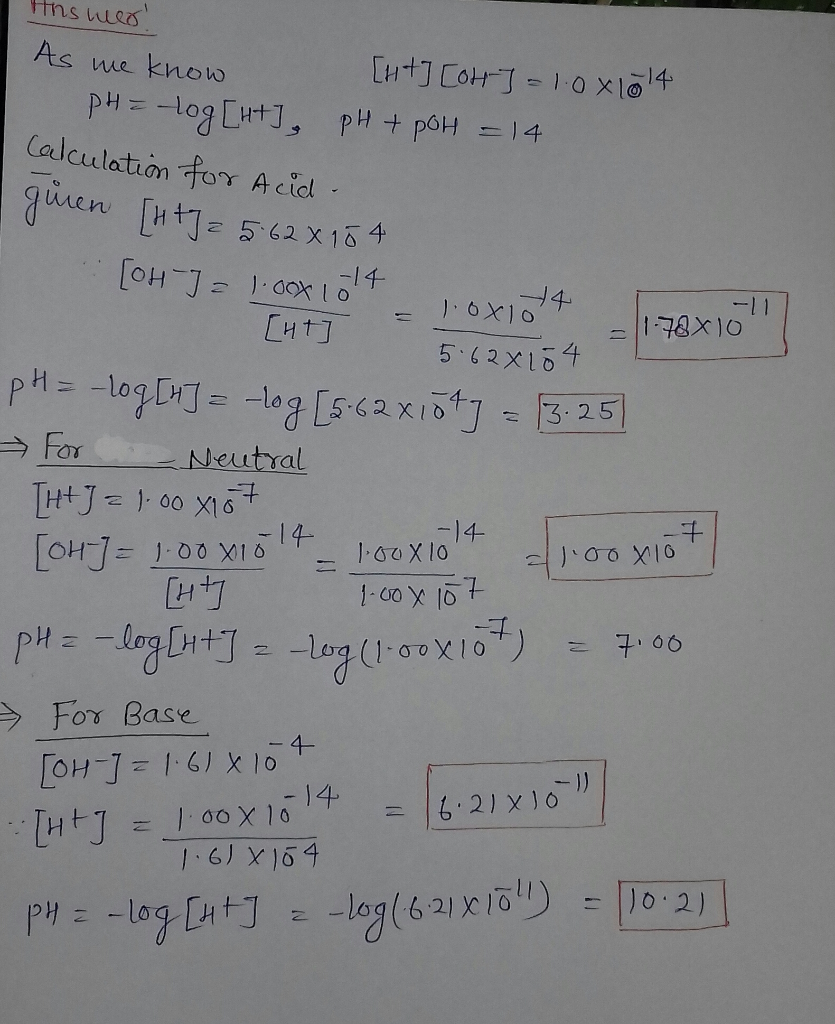#### Earn Coins

Coins can be redeemed for fabulous gifts.

Similar Homework Help Questions
• ### NAME: 1 Fill in the blanks with the appropriate value. Please express concentration values to 3...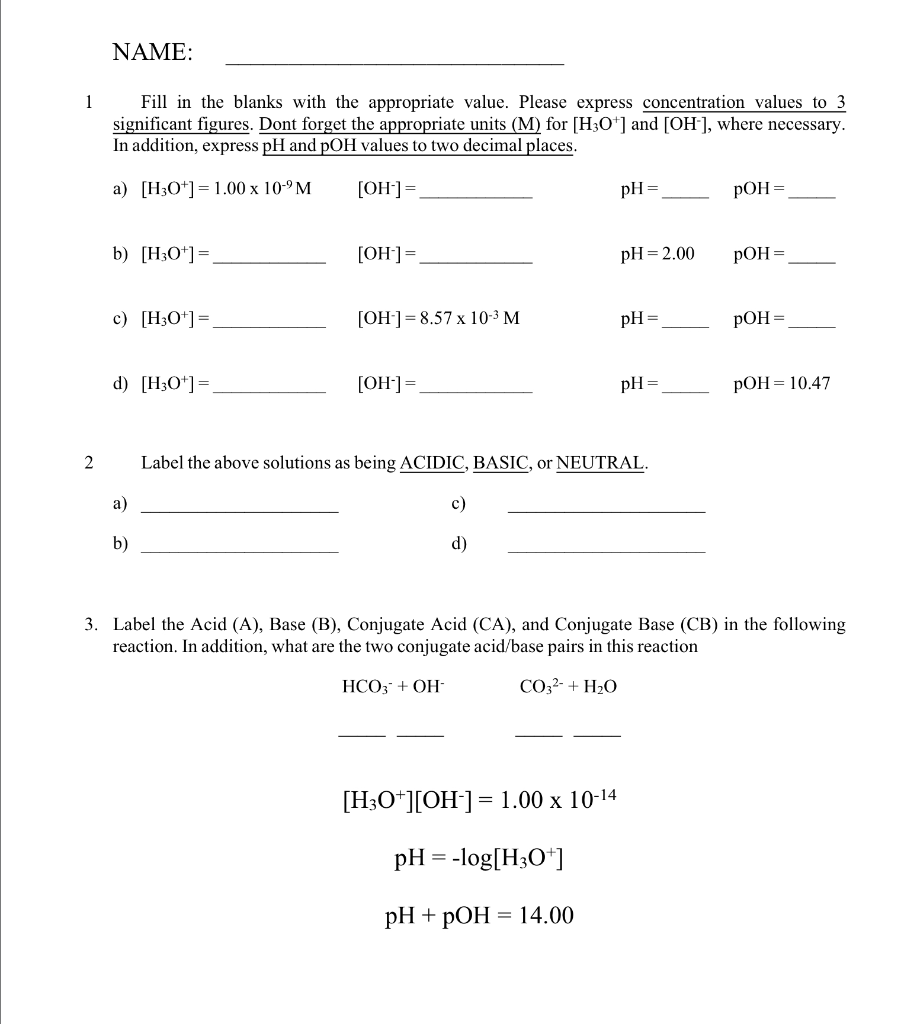NAME: 1 Fill in the blanks with the appropriate value. Please express concentration values to 3 significant figures. Dont forget the appropriate units (M) for [H3O+] and [OH-], where necessary. In addition, express pH and pOH values to two decimal places. a) [H3O+] = 1.00 x 10-'M [OH-]= pH = POH= b) [H3O+]= [OH-]= pH=2.00 POH= c) [H3O+]= [OH-] = 8.57 x 10-3 M pH = pOH = d) [H3O+]= [OH-]= pH= POH= 10.47 2 Label the above solutions as...

• ### Instructions: Complete all the problems in this assignment Show all your work and submit a PDF...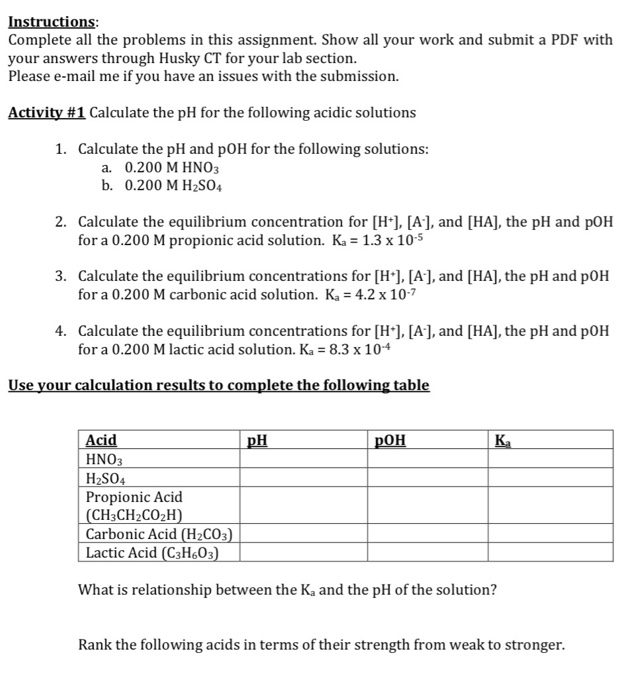Instructions: Complete all the problems in this assignment Show all your work and submit a PDF with your answers through Husky CT for your lab section. Please e-mail me if you have an issues with the submission. Activity #1 Calculate the pH for the following acidic solutions 1. Calculate the pH and pOH for the following solutions: a. 0.200 M HNO3 b. 0.200 M H2SO4 2. Calculate the equilibrium concentration for [H], [A-], and [HA], the pH and pOH for...

• ### Fill in the missing information in the following table. [H+] [OH-] PH POH Solution a 9.63...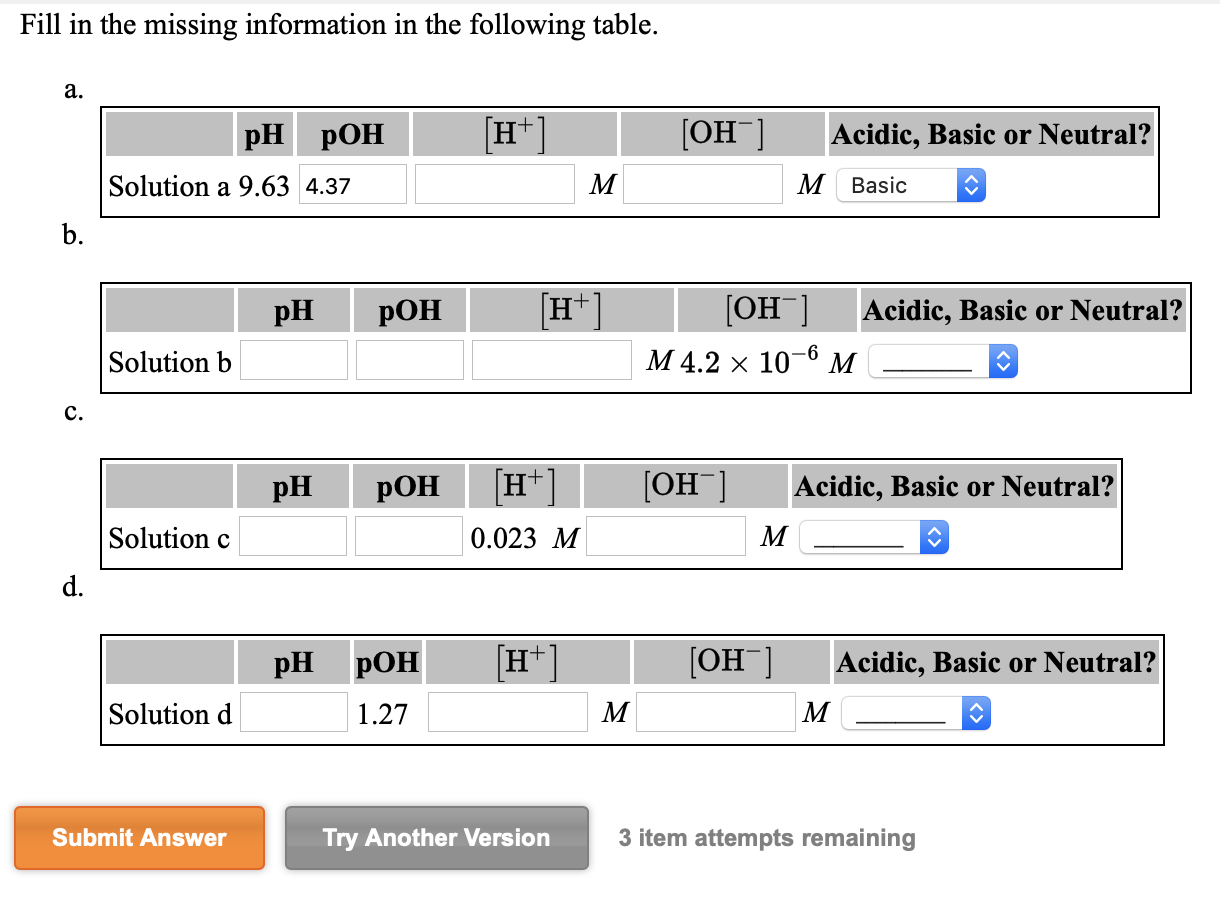Fill in the missing information in the following table. [H+] [OH-] PH POH Solution a 9.63 4.37 Acidic, Basic or Neutral? Basic M pH pOH (H+ OH-1 M 4.2 x 10-6 M Acidic, Basic or Neutral? - Solution b pH POH [OH-] H+] 0.023 M Acidic, Basic or Neutral? - Solutionc M pH POH H+1 [OH-] Acidic, Basic or Neutral? - Solution d 1.27 M _ M Submit Answer Try Another Version 3 item attempts remaining

• ### CHEMWORK For solutions of the same concentration, as acid strength increases [H] pH [OH-] POH Fill...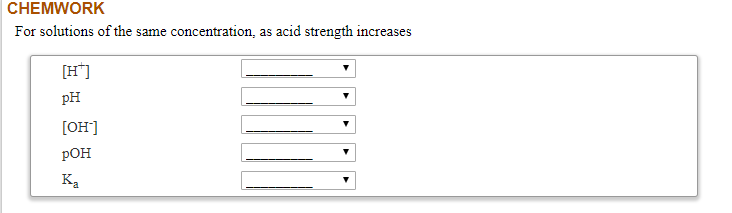CHEMWORK For solutions of the same concentration, as acid strength increases [H] pH [OH-] POH Fill in the missing information in the following table. POH [H+] [OH^] Acidic, Basic or Neutral? PH Solution a 9.61 pH POH [H+] Acidic, Basic or Neutral? [OH-] M4.2 x 10-6 M Solution b pH POH [OH-] Acidic, Basic or Neutral? [H+] 0.031 M Solution pH POH 1.23 (H+] M [OH-] M Acidic, Basic or Neutral? ___ Solution d

• ### Fill in the missing information in the following table. н он pH pOH Acidic, Basic or...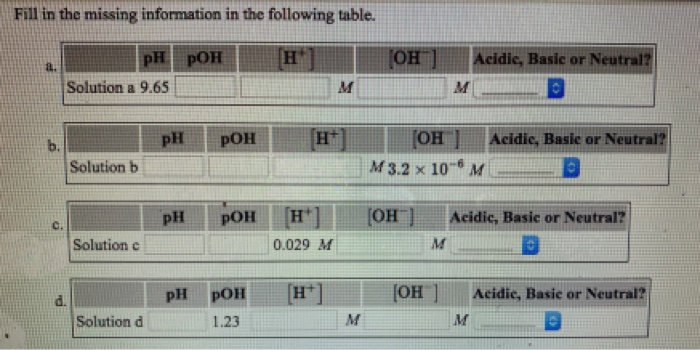Fill in the missing information in the following table. н он pH pOH Acidic, Basic or Neutral? Solution a 9.65 м [он M 3.2 x 10 H pH pOH Acidic, Basic or Neutral? b. Solution b M H (OH pH РОН Acidic, Basic or Neutral? Solution c M 0.029 M H*] [Он Acidic, Basic or Neutral? pOH pH Solution d м M 1.23

• ### Hello there. I need help with working on some calculations for two vials (with numbers 3...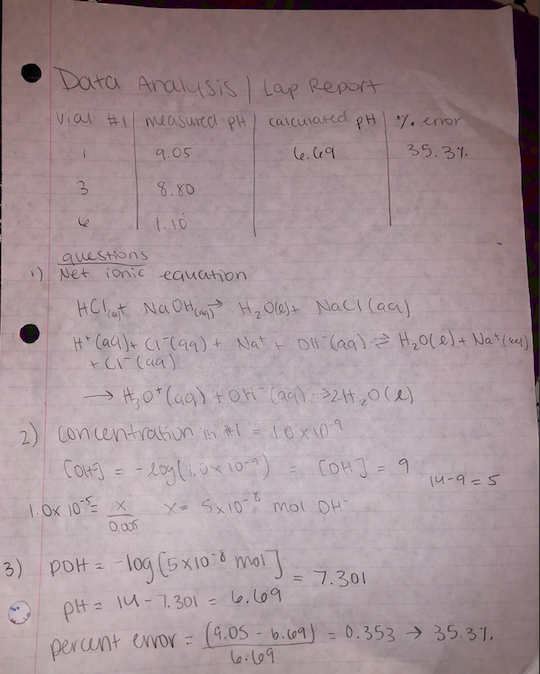Hello there. I need help with working on some calculations for two vials (with numbers 3 and 6). We did a lab that measured three vials. In vial #1, it had a pH of 9, 5 mL of solution was added, and the moles were 5x10^-8 mol OH-. The actual pH was 6.69 for vial #1. Calculate the number of moles of either OH- or H3O+ in the solution that was added. (OH-) if the solution was a base or...

• ### how would I fill out the table for acid base reactions in a solution [H:04) [OH-]...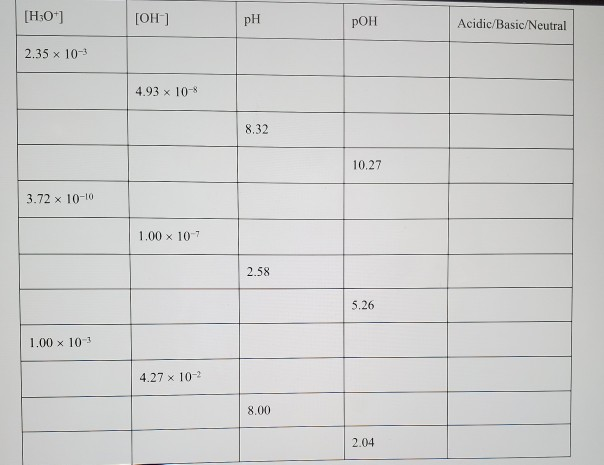how would I fill out the table for acid base reactions in a solution [H:04) [OH-] pH POH Acidic/Basic/Neutral 2.35 x 10-3 4.93 x 10- 8.32 10.27 3.72 x 10-10 1.00 x 107 2.58 1.00 x 10-3 4.27 x 10-2 8.00

• ### 1. Write the dissociation reaction and the corresponding K, equilibrium expression for each of the following...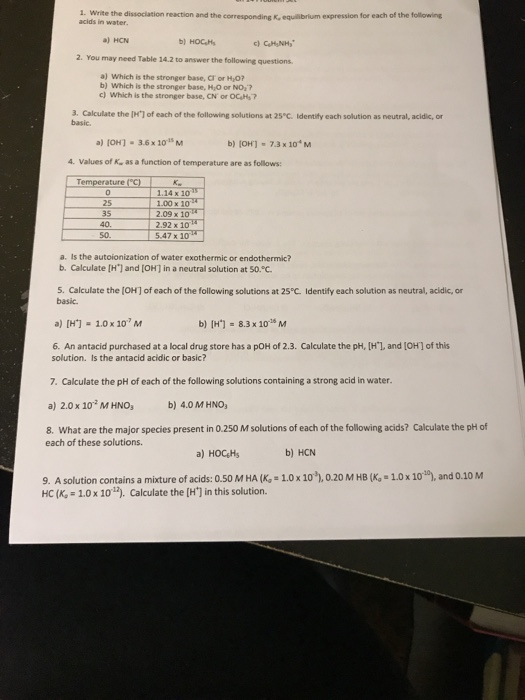1. Write the dissociation reaction and the corresponding K, equilibrium expression for each of the following )HCN bHOC.H 2. You may need Table 14.2 to answer the following questions a) Which is the stronger base, CT or HO b) Which is the stronger base, HiO or NO? c) which is the stronger base, Cr or OCH,7 3. Calculate the [H"1 of each of the following solutions at 25 C. Identity each solution as neutral, acidic, or basic. a) [OH] ....

• ### How do you get the 1.18 when doing the quadratic equation? Therefore, we have pH =...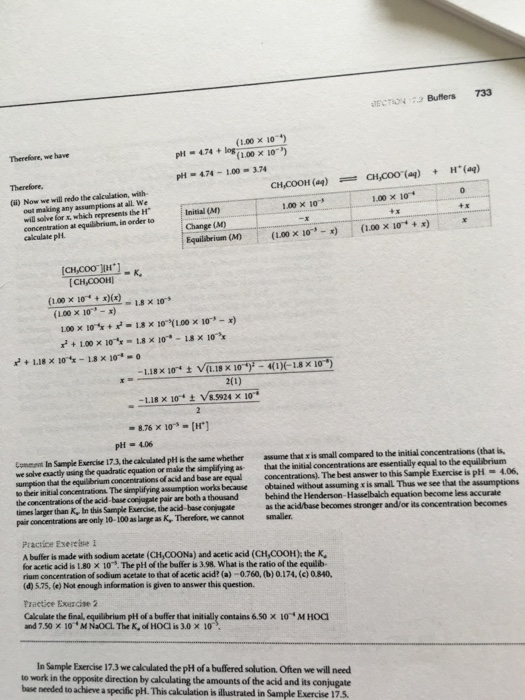How do you get the 1.18 when doing the quadratic equation? Therefore, we have pH = 4.74 + log(1.00 times 10^-4)/(1.00 times 10^-3) pH = 4.74 - 1.00 = 3.74 Therefore Now we will redo the calculation, without making any assumptions at all. We will solve for x, which represents the H^+ concentration at equilibrium, in order to calculate pH. [CH_3COO^-][H^+]/[CH_3COOH] = K_a (1.00 times 10^-4 + X)(X)/(1.00 times 10^-3 - X) = 1.8 times 10^-5 1.00 times 10^-4 X...

• ### Calculate and fill in the following information and indicate whether the solution is acidic, 4 basic,...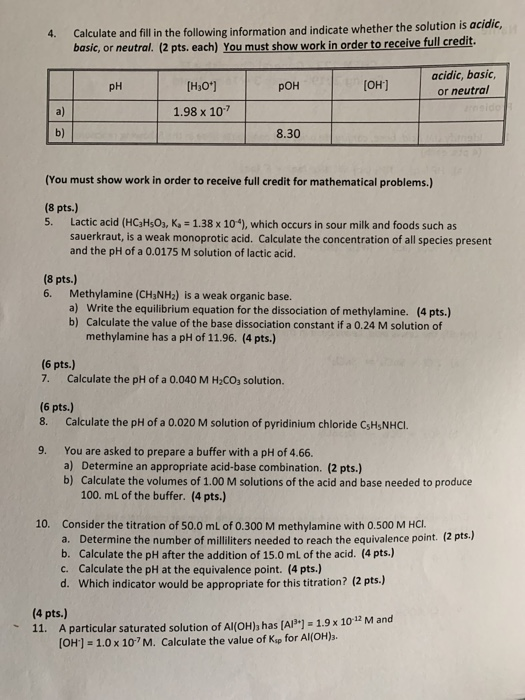Calculate and fill in the following information and indicate whether the solution is acidic, 4 basic, or neutral. (2 pts. each) You must show work in order to receive full credit. acidic, basic, or neutral [H3o] OH] pH POH 1.98 x 10 a) b) 8.30 (You must show work in order to receive full credit for mathematical problems.) (8 pts.) 5. Lactic acid (HCaHs03, K 1.38 x 10"), which occurs in sour milk and foods such as sauerkraut, is a...